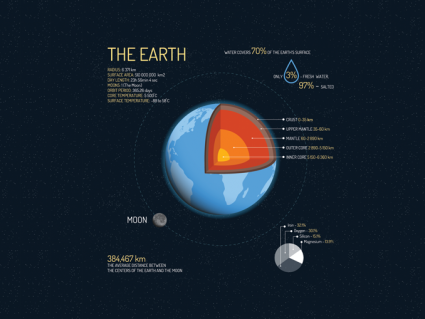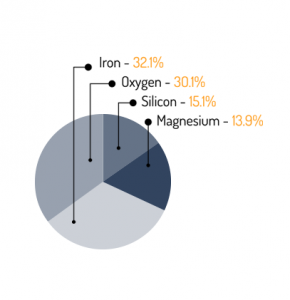# EARTH DENSITY: What is the Earth Made Of?

Last Updated: March 19, 2019

### Earth is the densest planet in the solar systemEarth density steadily increases as you go inwards from the crust to core. If you average it throughout the whole planet, Earth density is about 5.513 g/cm3.

It’s the largest and densest of all terrestrial planets. Terrestrial planets like Mercury, Venus, Earth and Mars tend to be rich in metals and silicate rocks.

If you crunch the numbers, Earth is mostly iron (35%), oxygen (30%), silicon (15%) and magnesium (13%). Finally, nickel (2%), sulfur (2%), calcium (1%) and aluminum (1%) make up most of the remaining.

So now we know what Earth is made of in terms of its composition. What is Earth density? And how do you calculate how dense Earth is?

### Earth density by core, mantle and crustIf you average density throughout the whole planet, then Earth density is about 5.513 g/cm3. But if you compare Earth density by its layers, density steadily increases as you go inwards from the crust to core.

For example, Earth’s inner core has the highest density at 12.9 g/cm3. Next, the outer core has a density of about 11.0 g/cm3.

The lower mantle has a significantly lower density of about 5.0 g/cm3. This is followed by the upper mantle with a density of 3.9 g/cm3.

Finally, the crust consists of rocks rich in silica with a density of about 2.5 g/cm3. The crust rides on a plasticky layer called the asthenosphere. This fluid-like layer with a density of about 3.3 g/cm3.

### How to calculate Earth density by hand

Density is mass divided by volume. Mass is the physical matter of something in total number of atoms. And volume is the amount of space an object encloses. If you know the mass and volume of an object, then you can measure density by dividing the two.

For example, Earth is not a perfect sphere. But we can approximate come to by using the volume of a sphere which is 4/3πr3. Based on a radius of 6,371 km, Earth’s volume would be 1.08 trillion cubic kilometers.

Given the mass of Earth is 5.972 × 10^24 kg, we can divide by its volume as noted above. After converting kilograms and cubic kilometers to grams and cubic centimeters, Earth density is about 5.519 g/cm3.

And this roughly checks in with the general consensus of Earth density at about 5.513 g/cm3. If you account for Earth shape, you can get a better estimate of Earth density.